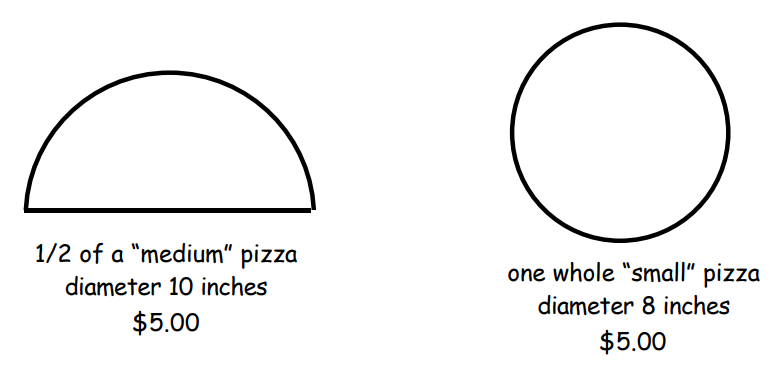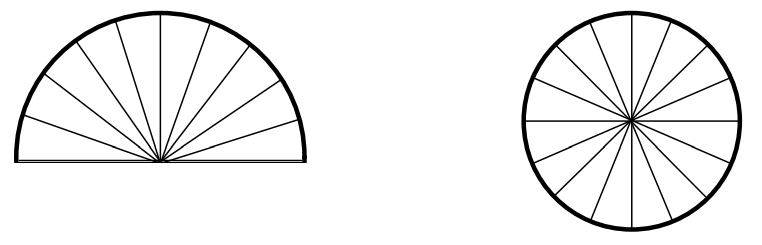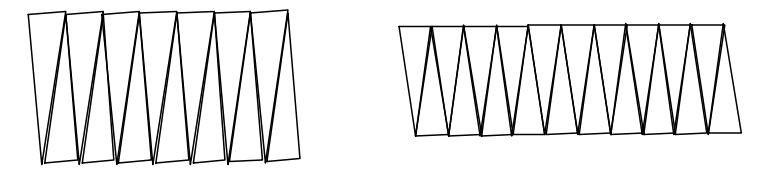Subject:
Mathematics

Topics: Geometry (Circles)Problem Solving
Common Core State Standard: 7.G.4,
Concepts:

· Area
· Diameter
· Circumference

Knowledge and Skills:

· Can determine the area of a rectangle by computation
· Can use the problem-solving strategy “make a model”
· Can determine the area of a circle as the product of the radius and half of the circumference (optional)

Materials:

(for each team):
· A drawing compass

Lesson:

Procedure: This activity can be done by students individually or in teams of two.

Distribute the handout, and ask someone in the class to describe the problem. At this point it is a good idea to discuss just what is meant by “bigger”. A larger diameter? Help students to see that what they would want is to choose the selection that gives them the most pizza, and that this depends on the area (the space inside a flat figure). This is a good place to review the concept of area and, while doing so, to remind students of the formula for the area of a rectangle.

You can at this point discuss problem solving strategies, leading up to the fact that the strategy they are asked to use in this problem is to make a model.

In order to make models with a compass, students will need to use the fact that the radius (the spread between the compass points) is half the diameter.

After the models are made, but before the next step, discuss the remainder of the procedure with the students, and in particular discuss whether or not cutting up their models and rearranging the pieces will change their areas. Be sure to have students explain their reasoning for their answers to this. If they do not clearly grasp that the areas would not change, demonstrate a simpler example by cutting a single rectangle sheet of paper in half and re-arranging it.

Before students cut their slices, have them draw lines for the cuts, using a ruler. It is important that the lines are straight and go exactly through the centers of the circles.

Check this before allowing students to make their cuts.

Once students have cut the slices and re-arranged them, you can have them tape the slices together in the new arrangement so that they hold their shape. Then students can measure the lengths and widths directly.

If the slices are thin enough, the shapes will be very close to rectangular. If not, they will be more like parallelograms. In that case (unless your students know how to compute the areas of parallelograms), you can change the shape into something much closer to a rectangle by cutting the slice on one end in half (vertically), and shifting the outside half to the other end.

Extension:

You can extend this by having students do these additional steps:

5) Enclose the second figure of step 3 in a rectangle, making the tightest fit possible. How do the area of the circle and the area of the rectangle compare? The area of the circle is a little less than the area of the rectangle.

6) How wide is the rectangle? What measurement on the circle is approximately the same height? The radius of the circle has about the same measure as the width of the rectangle.

7) How long is the rectangle? What part of the circle would have about the same measurement? Half the circumference is about the same measure as the length of the rectangle.

8) How can you use the measure of the parts of the circle you found in steps 6 and 7 to estimate the area of the rectangle? Multiply them.

9) How would your estimate change if you cut the paper “pizza” into thinner slices? How do the area of the circle and the area of the rectangle compare? The areas would be closer.

10) Explain how you might estimate the area of the whole circle if you knew the radius and the circumference of the circle. Multiply the radius by the one half of the circumference.

(Note: Since the circumference is equal to 2πr, then the area is equal to ½ x 2πr x r = πr x r = πr²)

### Which Pizza?

These two pizzas cost the same amount:Which one is bigger? Here is one way to find out.

1. Make a model of each pizza from paper, using a compass to draw the circles.

2. Cut each paper “pizza” into thin slices.3. Arrange the pieces to make shapes that are almost rectangles.4. Use what you know about rectangles to estimate the areas of the two pizzas. Which one is larger?